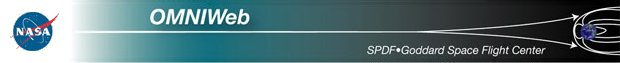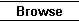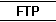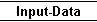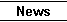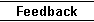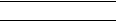Interface for Linear Regression Fits (LRF), Correlation Coefficients (CC) and scatter plots for single lag, and for Auto-CC or Cross-CC for series (up to 10) of lags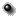All about using this interfaceSpecify Lag Number N: from 0(no lag) to 60;
[N is number of data points by which Y lags X]Select one of three option below
 LRF and scatter plot for lag=N List data selected for lag=N LRFs for lags from 0 to N with step M:  (M >= N/10)           [E.g., use M=3 for CC's at lags of 0, 3, 6, ... hours]Enter start and stop dates(Use yyyyddd or yyyymmdd)
Start      StopSelect any one or two variable(s) as X and Y axes
 Variable Name X/Y Axes Format Optional values:Low    High IMF Magnitude Avg, nT X    Y F5.1 Magnitude, Avg. IMF Vr, nT X    Y F5.1 Lat. of Avg. IMF, deg. X    Y F5.1 Long. of Avg. IMF, deg. X    Y F5.1 Bx, GSE/GSM, nT X    Y F5.1 By, GSE, nT X    Y F5.1 Bz, GSE, nT X    Y F5.1 By, GSM, nT X    Y F5.1 Bz, GSM, nT X    Y F5.1 Sigma in IMF Magnitude Avg. X    Y F5.1 Sigma in IMF Vector Avg X    Y F5.1 Sigma Bx, nT X    Y F5.1 Sigma By, nT X    Y F5.1 Sigma Bz, nT X    Y F5.1 Proton Temperature, K X    Y F8.0 Proton Density, n/cc X    Y F5.1 Flow Speed, km/sec X    Y F5.0 Flow Longitude, deg. X    Y F5.0 Flow Latitude, deg. X    Y F5.0 Alpha/Proton Density Ratio X    Y F5.3 Flow Pressure, nPa X    Y F5.1 Sigma-T X    Y F5.1 Sigma-Np X    Y F5.1 Sigma-V X    Y F5.1 Sigma-Flow-Longitude X    Y F5.1 Sigma-Flow-Latitude X    Y F5.1 Sigma-Alpha/Proton Ratio X    Y F5.1 Ey, Electric Field, mV/m X    Y F6.2 Plasma Beta X    Y F6.2 Alfven Mach number X    Y F5.1 Magnetosonic Mach number X    Y F5.1 Proton Quazy-Invariant X    Y F5.1 Kp*10 Index X    Y I2 R (Sunspot N#) X    Y I3 Dst Index, provisional when? X    Y I5 ap Index, nT X    Y I4 Solar index F10.7 X    Y F6.1 AE Index, provisional when? X    Y I4 AL Index, nT X    Y I5 AU Index, nT X    Y I5 PCN index from Thule X    Y F6.1 Proton Flux* > 1 MEV X    Y F9.2 Proton Flux* > 2 MEV X    Y F8.2 Proton Flux* > 4 MEV X    Y F8.2 Proton Flux* >10 MEV X    Y F8.2 Proton Flux* >30 MEV X    Y F8.2 Proton Flux* >60 MEV *1/(cm**2-sec-ster) X    Y F8.2 ap Index, nT X    Y I4 Solar index F10.7 X    Y F6.1 PC index from Thule X    Y F6.1 AL Index, nT provisional when? X    Y I5 AU Index, nT provisional when? X    Y I5 Lyman Alpha Solar index X    Y F9.6Select the fitting method
No fitting     Delta-Y method(N<30000)Advanced plot selections (optional)
 View Mode: Create inline GIF output Spawn external GIF viewer PostScript/Landscape PostScript/Portrait Character size(0.5-2.0): Plot Symbol: None Plus Asterisk Period Diamond Triangle Square X Symbol Size(0.1-4.0): Image size (pixels): X: Y: Connect Type: Connect data points Show points only

 SPDF > OMNIWeb

 If you have any questions/comments about OMNIWEB system, contact: Dr. Natalia Papitashvili, Space Physics Data Facility, Mail Code 672, NASA/Goddard Space Flight Center, Greenbelt, MD 20771